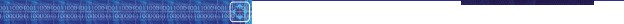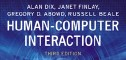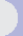HCI book > exercises > 1. human > ex. 1.1 text onlyexercises1. the humanEXERCISE 1.1

Devise experiments to test the properties of (i) short-term memory (ii) long term memory, using the experiments described in this chapter to help you. Try out your experiments on your friends. Are your results consistent with the properties described in this chapter?

The aim of this exercise is to get the student to think about experimental design. The experiments devised can effectively be repeats of the originals. Chapter 9 can be used for reference on experimental design.

(i) Short term memory (STM)

The student should first choose an aspect to investigate: for example, digit span, recency effect, decay.

Example solution: STM decay

Subjects
ideally selected to represent population, more probably undergraduate students (try to get a range of academic subjects).
Sample size: 10+
Experiment
• split subjects into two groups. Each subject studies list of 15-20 words (could try with both nonsense words and actual words to see any difference). Subject has to recall list either (a) immediately or (b) after 20 second delay. Measure the number (or percentage) of the words remembered correctly.
• A within-groups design can be used to avoid individual bias or group variation (as long as different lists are used for each attempt).
• independent variable -- delay in recall
dependent variable -- number correctly recalled.
• Group (b) should be given a task to do during the delay period in order to avoid rehearsal. If possible this task should occupy a different channel to minimise interference, e.g. a visual recognition task.
Hypothesis
Those in (b) will perform worse than those in (a) since STM will decay.
Analysis
graphs to see decay.
T test

(ii) Long term memory (LTM)

The student should first choose an aspect to investigate: for example, the total time hypothesis or the distribution of practice effect.

Example solution: distribution of practice effect

Subjects
as above. Should have no prior experience of the skill to be learned.
Experiment
• split subjects into 3 groups. Each subject must learn a skill (for example shorthand or typing -- must be measurable). Group A learns for 1 hour a week for 6 weeks. Group B learns for 2 hours a week for 3 weeks. Group C learns for 3 hours a week for 2 weeks.
• After each group's training is complete the subjects are tested and the number of mistakes made are noted.
• Between-groups design.
• independent variable - style of learning
dependent variable - accuracy
Hypothesis
Group A will be best (due to the distribution of practice effect)
Analysis
ANOVA

(N.B. This one is not easy to run but could be done with cooperation from friends)

 Other exercises in this chapter ex.1.1 (ans), ex.1.2 (ans), ex.1.3 (ans), ex.1.4 (tut), ex.1.5 (tut), ex.1.6 (open), ex.1.7 (open)all exercises for this chapter